# How To Use This Package

#### 2023-08-07

This example is inspired after the visualizations from Hausmann et al. (2014) with some ggplot additions. The original vignette was largely improved from what I learned in Network Analysis taught at ICPSR 2023 by Dr. Sarah Shugars.

# World Trade and Per-Capita GDP

``````library(economiccomplexity)

# partial view of trade matrix
``````##   country product value
## 1     afg    0011 30068
## 2     afg    0012 16366
## 3     afg    0111 19273
## 4     afg    0112   893
## 5     afg    0113   350
## 6     afg    0116  1561``````
``````# partial view of gdp vector
``````##   country value
## 1     abw 19185
## 2     ago  1540
## 3     alb  1433
## 4     and 27765
## 5     arb  3312
## 6     are 43082``````

# Balassa Index

You can obtain Balassa Index with `balassa_index()`.

``````bi <- balassa_index(world_trade_avg_1998_to_2000)

# partial view of index
bi[1:5, 1:5]``````
``````## 5 x 5 sparse Matrix of class "dgCMatrix"
##     0011 0012 0013 0014 0015
## afg    .    .    .    .    .
## ago    .    .    .    .    .
## aia    .    .    .    .    .
## alb    .    .    .    .    .
## and    1    .    .    .    1``````

Another possibility is to obtain Balassa Index without discretization.

``````bi_dec <- balassa_index(world_trade_avg_1998_to_2000, discrete = F)

# partial view of index
bi_dec[1:5, 1:5]``````
``````## 5 x 5 sparse Matrix of class "dgCMatrix"
##          0011        0012        0013       0014        0015
## afg 0.2312238 0.285438688 .           .          .
## ago 0.1777917 0.087436319 0.002076625 0.00139056 0.001106041
## aia .         0.002581853 .           .          .
## alb .         .           .           0.01582754 0.073011368
## and 2.4825815 0.550954168 .           0.48208017 3.815013019``````

# Complexity Measures

You can compute complexity indexes (e.g. such as the Economic Complexity Index and Product Complexity Index) by using `complexity_measures()`. The calculations methods are fitness (default), reflections, eigenvalues. See (Mariani et al. 2015) for the methodological details.

The eigenvalues also calls the reflections methods in order to correct the index sign in some special cases when the correlation between the output from both methods is negative.

## Complexity-Fitness

``````com_fit <- complexity_measures(bi)

# partial view of indexes
com_fit\$complexity_index_country[1:5]``````
``````##        afg        ago        aia        alb        and
## 0.78605655 0.03999516 1.05645538 1.24261128 1.37321261``````
``com_fit\$complexity_index_product[1:5]``
``````##      0011      0012      0013      0014      0015
## 0.7538883 0.7491391 2.3018690 0.9903918 1.3120378``````

## Reflections

``````com_ref <- complexity_measures(bi, method = "reflections")

# partial view of indexes
com_ref\$complexity_index_country[1:5]``````
``````##        afg        ago        aia        alb        and
## -0.5788151 -1.7710696  1.4074821 -0.1754989  1.0738736``````
``com_ref\$complexity_index_product[1:5]``
``````##        0011        0012        0013        0014        0015
## -0.66255107 -1.62169899 -0.07449487  0.20554720  0.15848845``````

## Eigenvalues

``````com_eig <- complexity_measures(bi, method = "eigenvalues")

# partial view of indexes
com_eig\$complexity_index_country[1:5]``````
``````##        afg        ago        aia        alb        and
## -0.5764283 -1.7770752  1.4090414 -0.1732606  1.0772452``````
``com_eig\$complexity_index_product[1:5]``
``````##        0011        0012        0013        0014        0015
## -0.66657613 -1.62657599 -0.08149436  0.19917280  0.14935653``````

# Proximity

Proximity matrices are used to create projections e.g. (country-country and product-product networks) for bipartite networks. Using `proximity()` is straightforward.

``````pro <- proximity(bi)

# partial view of proximity matrices
pro\$proximity_country[1:5, 1:5]``````
``````## 5 x 5 sparse Matrix of class "dsCMatrix"
##            afg         ago         aia        alb         and
## afg 1.00000000 0.015873016 0.181818182 0.19689119 0.192513369
## ago 0.01587302 1.000000000 0.006993007 0.01554404 0.005347594
## aia 0.18181818 0.006993007 1.000000000 0.16580311 0.251336898
## alb 0.19689119 0.015544041 0.165803109 1.00000000 0.310880829
## and 0.19251337 0.005347594 0.251336898 0.31088083 1.000000000``````
``pro\$proximity_product[1:5, 1:5]``
``````## 5 x 5 sparse Matrix of class "dsCMatrix"
##           0011      0012      0013      0014      0015
## 0011 1.0000000 0.3658537 0.1707317 0.2439024 0.2682927
## 0012 0.3658537 1.0000000 0.2500000 0.2250000 0.2500000
## 0013 0.1707317 0.2500000 1.0000000 0.2500000 0.1200000
## 0014 0.2439024 0.2250000 0.2500000 1.0000000 0.2250000
## 0015 0.2682927 0.2500000 0.1200000 0.2250000 1.0000000``````

# Projections

The `projections()` function is designed to use `igraph` for the internal computations and also to pass proximity-based networks to `igraph`, `ggraph` or export to Cytoscape by saving the output as csv/tsv.

``````library(igraph)

net <- projections(pro\$proximity_country, pro\$proximity_product)

# partial view of projections
E(net\$network_country)[1:5]``````
``````## + 5/484 edges from c486c2b (vertex names):
##  zaf--zwe tza--zmb tza--uga tuv--wlf tuv--umi``````
``E(net\$network_product)[1:5]``
``````## + 5/1505 edges from 5b966f9 (vertex names):
##  8981--8982 8946--9510 8922--8932 8921--8922 8852--8959``````

We can also use `igraph` to see how many edges are in the networks nd also the networks’ density, diameter and transitivity.

``ecount(net\$network_country)``
``##  484``
``ecount(net\$network_product)``
``##  1505``
``edge_density(net\$network_country, loops = F)``
``##  0.01903638``
``edge_density(net\$network_product, loops = F)``
``##  0.00489081``
``diameter(net\$network_country, directed = F, unconnected = F)``
``##  7.845477``
``diameter(net\$network_product, directed = F, unconnected = F)``
``##  12.78569``
``transitivity(net\$network_country, type = "global")``
``##  0.6151159``
``transitivity(net\$network_product, type = "global")``
``##  0.4457284``

# Centrality measures

We calculate the degree centrality of every node in the network and plot a histogram of these values. The drawback is that the network was trimmed until obtaining an average of 4 links per edge (or arcs per node), therefore the computation and histograms reflect a biased distribution.

``````deg_country <- degree(net\$network_country)
deg_product <- degree(net\$network_product)

# country with the highest degree centrality
deg_country[which.max(deg_country)]``````
``````## svn
##  25``````
``````# product with the highest degree centrality
deg_product[which.max(deg_product)]``````
``````## 8421
##   33``````

In the same way, we can compute the betweenness, cloness and eigenvector centrality of the networks.

``````bet_country <- betweenness(net\$network_country)
bet_product <- betweenness(net\$network_product)

clo_country <- closeness(net\$network_country)
clo_product <- closeness(net\$network_product)

eig_country <- eigen_centrality(net\$network_country)\$vector
eig_product <- eigen_centrality(net\$network_product)\$vector

# country with the highest betweenness centrality
bet_country[which.max(bet_country)]``````
``````##   ken
## 16718``````
``````# product with the highest betweenness centrality
bet_product[which.max(bet_product)]``````
``````##    7412
## 53858.5``````
``````# country with the highest closeness centrality
clo_country[which.max(clo_country)]``````
``````##         ken
## 0.002555277``````
``````# product with the highest closeness centrality
clo_product[which.max(clo_product)]``````
``````##         7412
## 0.0005298341``````
``````# country with the highest eigenvector centrality
eig_country[which.max(eig_country)]``````
``````## cze
##   1``````
``````# product with the highest eigenvector centrality
eig_product[which.max(eig_product)]``````
``````## 8434
##    1``````

Following the analysis, we can verify that the largest connected component is the same as the original networks in this case.

``````# sub-networks of the largest connected component

lcc_countries <- induced_subgraph(
net\$network_country,
which(components(net\$network_country)\$membership ==
which.max(components(net\$network_country)\$csize))
)

lcc_products <- induced_subgraph(
net\$network_product,
which(components(net\$network_product)\$membership ==
which.max(components(net\$network_product)\$csize))
)

# is this the same as the original networks?

ecount(lcc_countries) == ecount(net\$network_country)``````
``##  TRUE``
``vcount(lcc_countries) == vcount(net\$network_product)``
``##  FALSE``
``````deg2_countries <- degree(lcc_countries)
deg2_products <- degree(lcc_products)

bet2_countries <- betweenness(lcc_countries)
bet2_products <- betweenness(lcc_products)

clo2_countries <- closeness(lcc_countries)
clo2_products <- closeness(lcc_products)

eig2_countries <- eigen_centrality(lcc_countries)\$vector
eig2_products <- eigen_centrality(lcc_products)\$vector

all.equal(deg_country[which.max(deg_country)], deg2_countries[which.max(deg2_countries)])``````
``##  TRUE``
``all.equal(deg_product[which.max(deg_product)], deg2_products[which.max(deg2_products)])``
``##  TRUE``
``all.equal(bet_country[which.max(bet_country)], bet2_countries[which.max(bet2_countries)])``
``##  TRUE``
``all.equal(bet_product[which.max(bet_product)], bet2_products[which.max(bet2_products)])``
``##  TRUE``
``all.equal(clo_country[which.max(clo_country)], clo2_countries[which.max(clo2_countries)])``
``##  TRUE``
``all.equal(clo_product[which.max(clo_product)], clo2_products[which.max(clo2_products)])``
``##  TRUE``
``all.equal(eig_country[which.max(eig_country)], eig2_countries[which.max(eig2_countries)])``
``##  TRUE``
``all.equal(eig_product[which.max(eig_product)], eig2_products[which.max(eig2_products)])``
``##  TRUE``

# K-core and backbone

We can identify the k-core of the networks for an arbitray value “k”.

``````k <- 4

# identify the core of the network
core_country <- coreness(net\$network_country, mode = "all")
core_product <- coreness(net\$network_product, mode = "all")

# identify the nodes in the core
kcore_country <- induced_subgraph(
net\$network_country,
which(core_country >= k)
)

kcore_product <- induced_subgraph(
net\$network_product,
which(core_product >= k)
)

V(kcore_country)\$name``````
``````##   "aut" "bel" "bgr" "bih" "blr" "che" "chn" "cze" "deu" "dnk" "esp" "est"
##  "fin" "fra" "gbr" "grc" "hkg" "hrv" "hun" "ind" "ita" "jpn" "ken" "lbn"
##  "ltu" "mkd" "nld" "pak" "pol" "prt" "rom" "scg" "svk" "svn" "swe" "tur"
##  "ukr" "usa"``````
``V(kcore_product)\$name``
``````##    "0142" "0223" "0240" "0252" "0484" "0565" "0583" "0586" "0620" "0730"
##   "0980" "5332" "5334" "5335" "5417" "5419" "5542" "5543" "5821" "5825"
##   "5989" "6123" "6210" "6289" "6343" "6354" "6359" "6416" "6417" "6418"
##   "6421" "6422" "6424" "6428" "6518" "6546" "6575" "6581" "6583" "6584"
##   "6589" "6618" "6631" "6632" "6633" "6635" "6648" "6794" "6911" "6912"
##   "6921" "6924" "6940" "6954" "6973" "6975" "6991" "6996" "6997" "6998"
##   "7139" "7211" "7212" "7213" "7219" "7259" "7269" "7281" "7368" "7369"
##   "7371" "7372" "7412" "7413" "7416" "7423" "7429" "7432" "7436" "7439"
##   "7449" "7452" "7492" "7493" "7499" "7711" "7732" "7742" "7757" "7758"
##   "7783" "7810" "7821" "7849" "7868" "7919" "8121" "8122" "8211" "8212"
##  "8219" "8421" "8422" "8423" "8424" "8429" "8431" "8432" "8433" "8434"
##  "8435" "8439" "8441" "8442" "8443" "8451" "8452" "8459" "8461" "8462"
##  "8463" "8464" "8465" "8472" "8510" "8720" "8742" "8922" "8931" "8932"
##  "8939" "8997"``````

We can also identify the backbone of the networks.

``````# identify the backbone of the network
bbn_country <- delete_vertices(net\$network_country, which(core_country < k))
bbn_product <- delete_vertices(net\$network_product, which(core_product < k))

bbn_country``````
``````## IGRAPH f196441 UNW- 38 283 --
## + attr: name (v/c), weight (e/n)
## + edges from f196441 (vertex names):
##   swe--usa svn--usa svn--tur svn--swe svk--usa svk--swe svk--svn scg--ukr
##   scg--tur scg--svn scg--svk rom--ukr rom--tur rom--svn rom--svk rom--scg
##  prt--tur prt--svn prt--svk prt--scg prt--rom pol--usa pol--tur pol--swe
##  pol--svn pol--svk pol--scg pol--rom pol--prt pak--tur nld--usa nld--swe
##  nld--svk mkd--tur mkd--scg mkd--rom mkd--prt mkd--pak ltu--ukr lbn--tur
##  lbn--mkd ken--tur ken--scg ken--lbn jpn--usa jpn--swe jpn--svn ita--usa
##  ita--tur ita--swe ita--svn ita--svk ita--prt ita--pol ita--nld ita--jpn
##  ind--tur ind--scg ind--rom ind--pak ind--ken ind--ita hun--usa hun--tur
## + ... omitted several edges``````
``bbn_product``
``````## IGRAPH 3ea69b8 UNW- 132 709 --
## + attr: name (v/c), weight (e/n)
## + edges from 3ea69b8 (vertex names):
##   8922--8932 8720--8939 8720--8742 8472--8997 8465--8510 8465--8472
##   8464--8472 8464--8465 8463--8510 8463--8472 8463--8465 8463--8464
##  8462--8472 8462--8465 8462--8464 8462--8463 8461--8472 8461--8465
##  8461--8463 8459--8472 8459--8465 8459--8464 8459--8463 8459--8462
##  8452--8472 8452--8465 8452--8464 8452--8463 8452--8462 8452--8461
##  8452--8459 8451--8997 8451--8472 8451--8465 8451--8464 8451--8463
##  8451--8462 8451--8459 8451--8452 8443--8997 8443--8472 8443--8465
##  8443--8464 8443--8463 8443--8462 8443--8459 8443--8452 8443--8451
## + ... omitted several edges``````

# Community detection

We can identify the communities of the networks with a fast greedy algorithm.

``````com_country <- cluster_fast_greedy(net\$network_country)
com_product <- cluster_fast_greedy(net\$network_product)

all.equal(vcount(net\$network_country), length(com_country\$membership))``````
``##  TRUE``
``all.equal(vcount(net\$network_product), length(com_product\$membership))``
``##  TRUE``
``````# number of communities
length(unique(com_country\$membership))``````
``##  12``
``length(unique(com_product\$membership))``
``##  41``

# Complexity Outlook

Both the Complexity Outlook Index and Complexity Outlook Gain are obtained after the `complexity_outlook()` function.

``````co <- complexity_outlook(
economiccomplexity_output\$balassa_index,
economiccomplexity_output\$proximity\$proximity_product,
economiccomplexity_output\$complexity_measures\$complexity_index_product
)

# partial view of complexity outlook
co\$complexity_outlook_index[1:5]``````
``````##        afg        ago        aia        alb        and
## 103.948610   9.962401 122.311158 152.107317 151.295380``````
``co\$complexity_outlook_gain[1:5, 1:5]``
``````## 5 x 5 Matrix of class "dgeMatrix"
##          0011      0012      0013      0014      0015
## afg 0.8615531 0.7613878 0.7537907 1.0961458 0.8143851
## ago 0.9681802 0.8436855 0.8219918 1.2151040 0.8883146
## aia 0.8339114 0.7475080 0.7247198 1.0425043 0.7671877
## alb 0.7979779 0.7199705 0.7132042 1.0170093 0.7636545
## and 0.0000000 0.7118512 0.6829531 0.9987236 0.0000000``````

# Productivy Levels

The `productivity_levels()` dataset follows the definitions from Hausmann et al. (2014) and Hausmann, Hwang, and Rodrik (2005).

I don’t have a per-capita GDP dataset for the Galactic Federation, so I’ll create simulated data for the example.

``````pl <- productivity_levels(world_trade_avg_1998_to_2000, world_gdp_avg_1998_to_2000)

# partial view of productivity levels
pl\$productivity_level_country[1:5]``````
``````##       ago       alb       and       are       arg
##  8223.607  6343.341 13783.485 10207.679  9269.670``````
``pl\$productivity_level_product[1:5]``
``````##      0011      0012      0013      0014      0015
##  7915.893  3986.371 11375.710  6273.428 17628.950``````

# Integration with `ggplot2`

We can plot the distributions for the centrality measures.

``````library(ggplot2)

deg_country <- data.frame(
country = names(deg_country),
deg = deg_country
)

deg_product <- data.frame(
product = names(deg_product),
deg = deg_product
)

ggplot(deg_country) +
geom_histogram(aes(x = deg), bins = 20, fill = "#002948") +
theme_minimal(base_size = 13) +
labs(title = "Degree Centrality Distribution for Countries")``````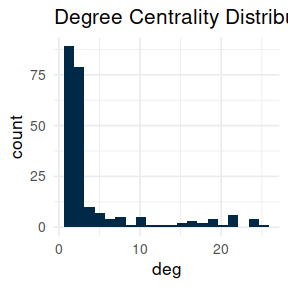``````ggplot(deg_product) +
geom_histogram(aes(x = deg), bins = 20, fill = "#002948") +
theme_minimal(base_size = 13) +
labs(title = "Degree Centrality Distribution for Products")``````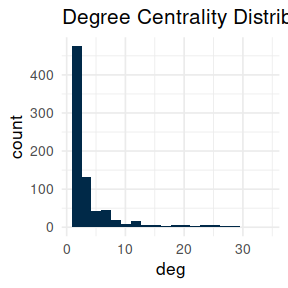``````bet_country <- data.frame(
country = names(bet_country),
bet = bet_country
)

bet_product <- data.frame(
product = names(bet_product),
bet = bet_product
)

clo_country <- data.frame(
country = names(clo_country),
clo = clo_country
)

clo_product <- data.frame(
product = names(clo_product),
clo = clo_product
)

eig_country <- data.frame(
country = names(eig_country),
eig = eig_country
)

eig_product <- data.frame(
product = names(eig_product),
eig = eig_product
)

ggplot(bet_country) +
geom_histogram(aes(x = bet), bins = 20, fill = "#002948") +
theme_minimal(base_size = 13) +
labs(title = "Betweenness Centrality Distribution for Countries")``````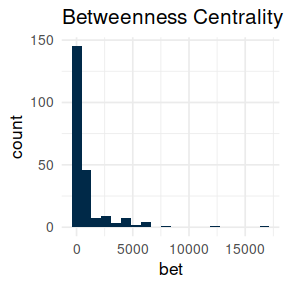``````ggplot(bet_product) +
geom_histogram(aes(x = bet), bins = 20, fill = "#002948") +
theme_minimal(base_size = 13) +
labs(title = "Betweenness Centrality Distribution for Products")``````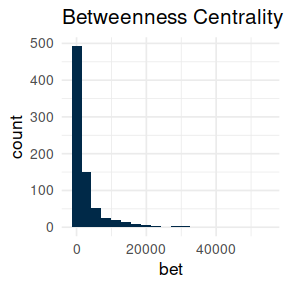``````ggplot(clo_country) +
geom_histogram(aes(x = clo), bins = 20, fill = "#002948") +
theme_minimal(base_size = 13) +
labs(title = "Closeness Centrality Distribution for Countries")``````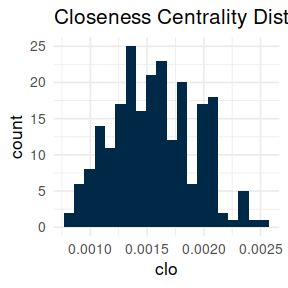``````ggplot(clo_product) +
geom_histogram(aes(x = clo), bins = 20, fill = "#002948") +
theme_minimal(base_size = 13) +
labs(title = "Closeness Centrality Distribution for Products")``````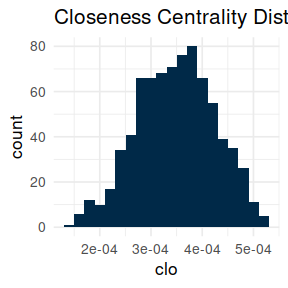``````ggplot(eig_country) +
geom_histogram(aes(x = eig), bins = 20, fill = "#002948") +
theme_minimal(base_size = 13) +
labs(title = "Eigenvector Centrality Distribution for Countries")``````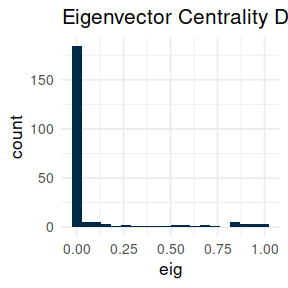``````ggplot(eig_product) +
geom_histogram(aes(x = eig), bins = 20, fill = "#002948") +
theme_minimal(base_size = 13) +
labs(title = "Eigenvector Centrality Distribution for Products")``````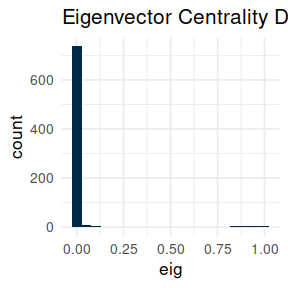# Integration with `ggraph`

We start by plotting the network of countries. Each node will be sized by its total exports.

``````set.seed(200100)

library(ggraph)

aggregated_countries <- aggregate(
FUN = sum
)

aggregated_countries <- setNames(aggregated_countries\$x, aggregated_countries\$country)

V(net\$network_country)\$size <- aggregated_countries[match(V(net\$network_country)\$name, names(aggregated_countries))]

ggraph(net\$network_country, layout = "kk") +
# geom_edge_link(aes(edge_width = weight), edge_colour = "#a8a8a8") +
geom_node_point(aes(size = size), color = "#002948") +
geom_node_text(aes(label = name), size = 2, vjust = 2.2) +
ggtitle("Proximity Based Network Projection for Countries") +
theme_void()``````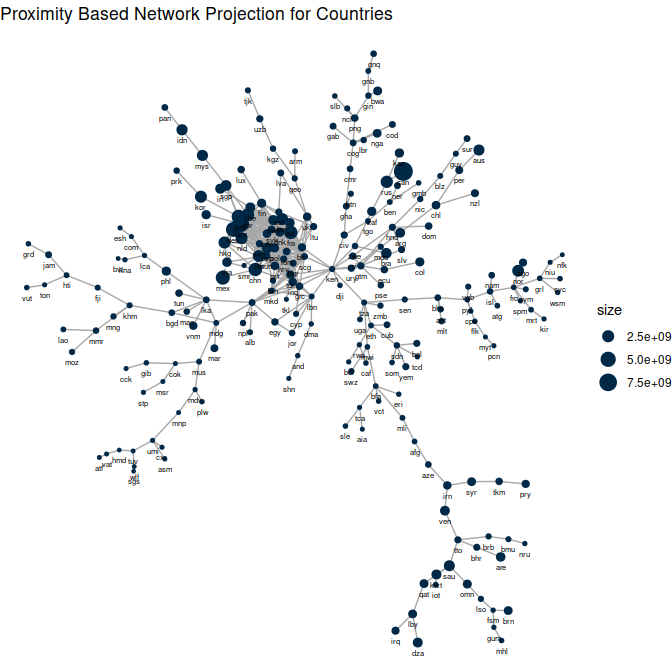Now we can highlight the countries with the highest centralities from the previous part.

``````# Paint svn, ken and cze in yellow and the rest of the world in blue

V(net\$network_country)\$color <- rep(
"Rest of the World",
length(V(net\$network_country)\$size)
)
V(net\$network_country)\$color[match(
c("svn", "ken", "cze"),
V(net\$network_country)\$name
)] <- "Slovakia, Kenia and Czech Republic"

ggraph(net\$network_country, layout = "kk") +
geom_node_point(aes(size = size, color = color)) +
geom_node_text(aes(label = name), size = 2, vjust = 2.2) +
ggtitle("Proximity Based Network Projection for Countries") +
theme_void() +
scale_colour_manual(values = c(
"Slovakia, Kenia and Czech Republic" = "#fac704",
"Rest of the World" = "#002948"
))``````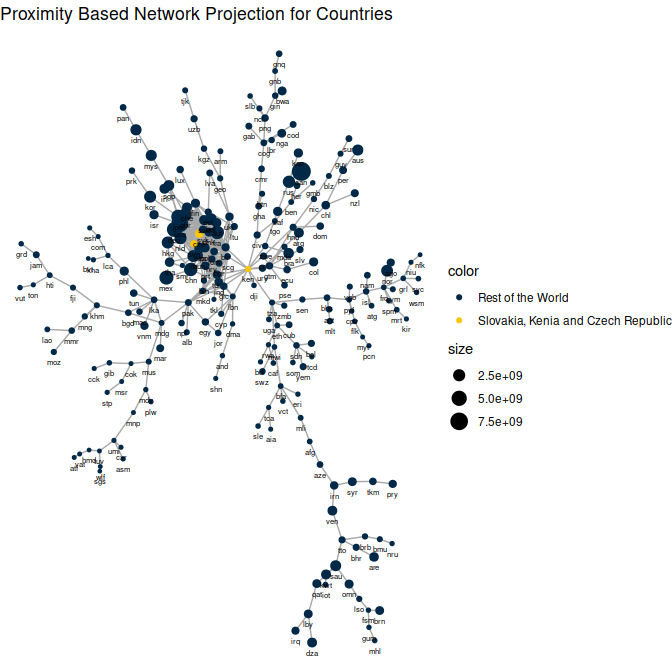We can also plot the network of products. Each node will be sized by its total exports. Because the product names are large, we display the product codes instead. You can read about the codes here and if you need the codes in R, you can use the `tradestatistics` package, which can be installed from CRAN.

``````set.seed(200100)

aggregated_products <- aggregate(
FUN = sum
)

aggregated_products <- setNames(aggregated_products\$x, aggregated_products\$country)

V(net\$network_product)\$size <- aggregated_products[
match(V(net\$network_product)\$name, names(aggregated_products))
]

ggraph(net\$network_product, layout = "kk") +
geom_node_point(aes(size = size), color = "#002948") +
geom_node_text(aes(label = name), size = 2, vjust = 2.2) +
ggtitle("Proximity Based Network Projection for Products") +
theme_void()``````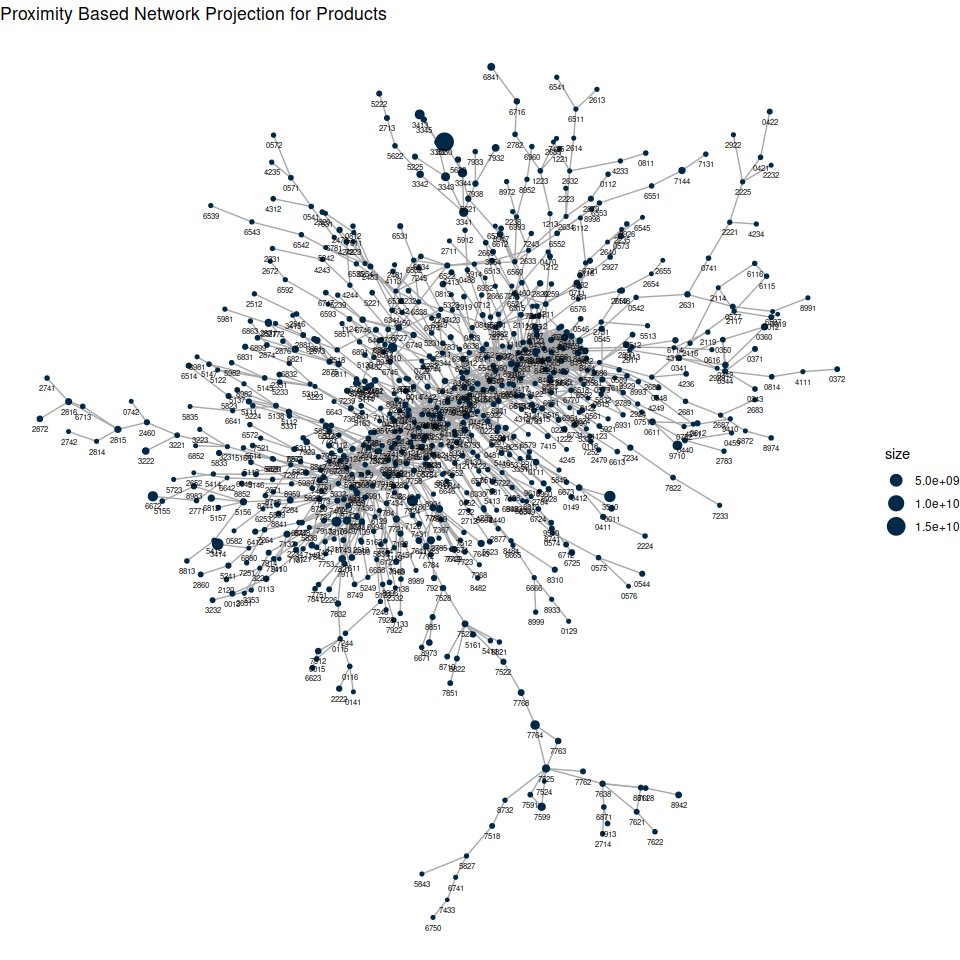Now we can highlight the products with the highest centralities from the previous part.

``````# Paint 8421, 7412 and 8434 in yellow and the rest of the products in blue

V(net\$network_product)\$color <- rep(
"Rest of the Products",
length(V(net\$network_product)\$size)
)
V(net\$network_product)\$color[match(
c("8421", "7412", "8434"),
V(net\$network_product)\$name
)] <- "8421, 7412 and 8434"

ggraph(net\$network_product, layout = "kk") +